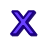Games
Problems
Go Pro!

# Multiplication Facts (All)

Quizzes > Mathematics > Arithmetic > MultiplicationPractice your multiplication tables with this quiz. Students who remember their multiplication facts work much more efficiently than students who rely on a calculator.

When you click Start Quiz, multiple-choice questions will appear one at a time. Choose the correct answers, and find out how much you know!
Quiz by Mr. TwitchellAssign this quiz# Blogs on This SiteReviews and book lists - books we love!The site administrator fields questions from visitors.

# Questions

Here are some of the questions you may encounter in the quiz Multiplication Facts (All).

9 × 8 = ?
5 × 6 = ?
1 × 5 = ?
2 × 1 = ?
3 × 7 = ?
3 × 2 = ?
3 × 1 = ?
9 × 2 = ?
5 × 8 = ?
9 × 0 = ?Like us on Facebook to get updates about new resources